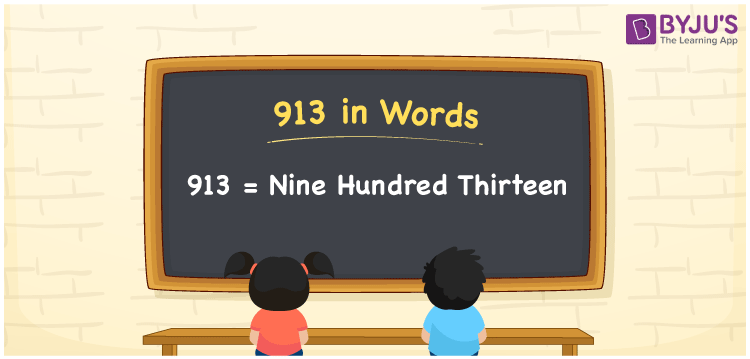# 913 in Words

913 in words is written as Nine Hundred Thirteen. The name of number 913 in English is “Nine Hundred Thirteen”. The word Nine Hundred Thirteen is used in many real-life situations. For example, there are Nine Hundred Thirteen students in a classroom. Thus, it is a counting number. Also, to represent the currency equal to 913, we can write it in words as Rupees Nine Hundred Thirteen or Nine Hundred Thirteen rupees. Therefore, it is necessary to learn the numbers in words, for the ease of understanding and expressing them.

 913 in words Nine Hundred Thirteen Nine Hundred Thirteen in Numbers 913

## 913 in English Words## How to Write 913 in Words?

If we know the place value of digits of 913, then we can easily express it in words. The place value is basically the position of a digit in a number. 913 is a three-digit number, therefore, we can specify the position of each digit of 913 in a place value chart. In the Indian numbering system, the order of place value of digits from right to left is given by:

 Hundreds Tens Ones 9 1 3

We can write it in expanded form as:

9 × Hundred + 1 × Ten + 3 × One

= 9 × 100 + 1 × 10 + 3 × 1

= 900 + 10 + 3

= 913

= Nine Hundred Thirteen

Therefore, 913 in words is written as eight hundred thirties.

913 is a natural number that precedes 914 and succeeds 912.

913 in words – Nine Hundred Thirteen

Is 913 an odd number? – Yes

Is 913 an even number? – No

Is 913 a perfect square number? – No

Is 913 a perfect cube number? – No

Is 913 a prime number? – No

Is 913 a composite number? – Yes

## Frequently Asked Questions on 913 in Words

### How to write 913 in words?

913 in English is written as “Nine Hundred Thirteen”.

### Is 913 an odd number or even number?

913 is an odd number because it is not wholly divisible by 2.

### Write Nine Hundred Thirteen in numbers.

Nine Hundred Thirteen in numbers is 913.